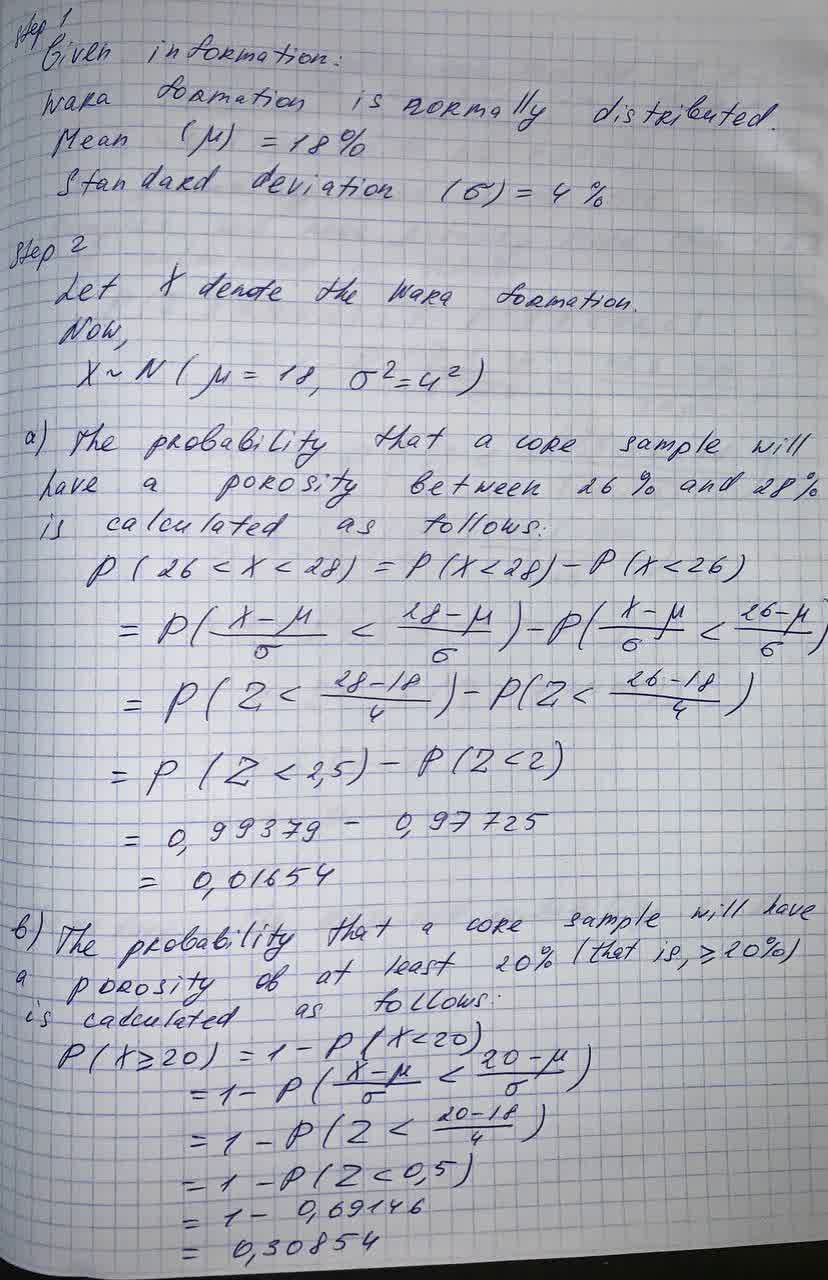# What is the probability that a core sample has a porosity of at least 20%?Reeves 2021-09-05 Answered
The porosity of Wara formation is normally distributed with a mean of 18% and a standard deviation of 4%.
What is the probability that a core sample has a porosity of at least 20%?
You can still ask an expert for help

## Want to know more about Probability?

• Questions are typically answered in as fast as 30 minutes

Solve your problem for the price of one coffee

• Math expert for every subject
• Pay only if we can solve itkrolaniaN
The answer is given below in the photo